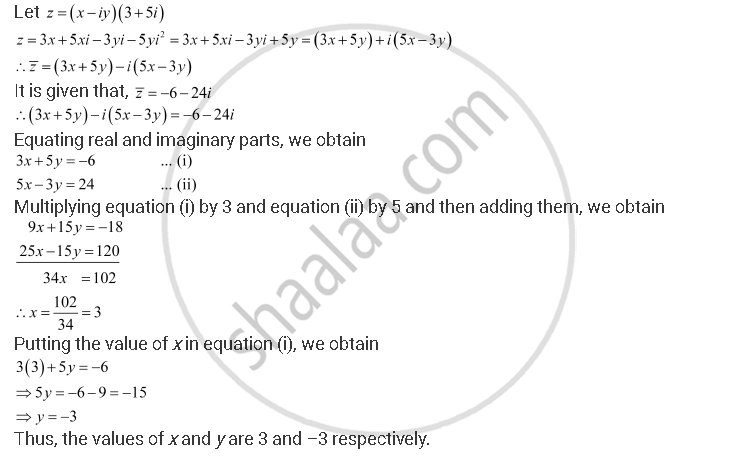CBSE (Arts) Class 11CBSE
Share

# Find the Real Numbers X and Y If (X – Iy) (3 + 5i) is the Conjugate of –6 – 24i. - CBSE (Arts) Class 11 - Mathematics

ConceptThe Modulus and the Conjugate of a Complex Number

#### Question

Find the real numbers x and y if (x – iy) (3 + 5i) is the conjugate of –6 – 24i.

#### SolutionIs there an error in this question or solution?

#### Video TutorialsVIEW ALL 

Solution Find the Real Numbers X and Y If (X – Iy) (3 + 5i) is the Conjugate of –6 – 24i. Concept: The Modulus and the Conjugate of a Complex Number.
S